# Spring stiffnesses vs. bar stiffness in FEM-Design and WIN-Statik

By using fictious bars in FEM-Design and selecting different stiffnesses on the bar it is possible to get different results of the resultants.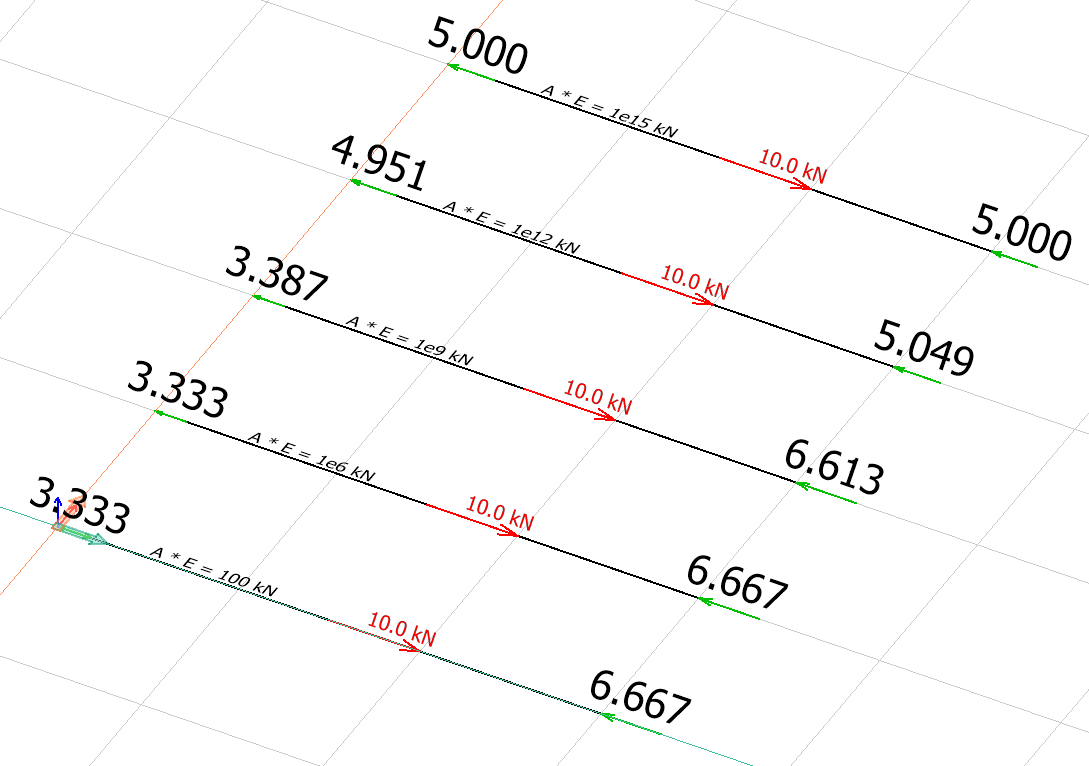In Frame Analysis it is possible to add different bars, and in this case we are keeping the Young modulus constant while changing the A (area):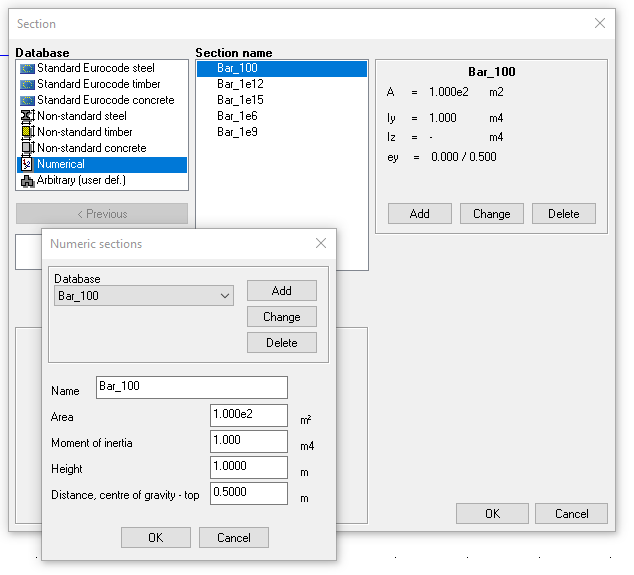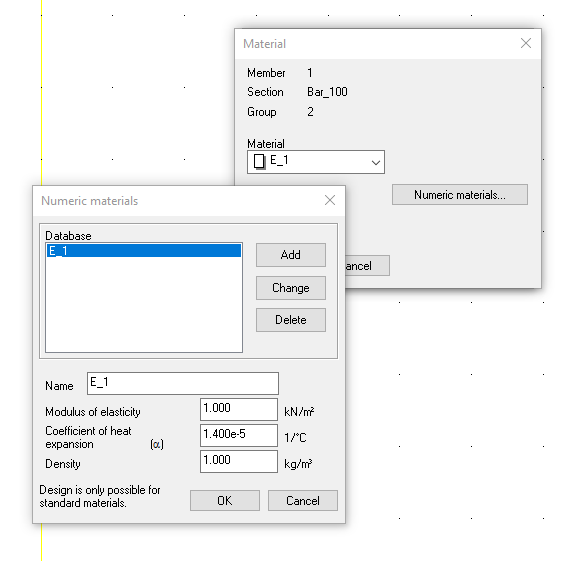So making a model in Frame Analysis would look like this with different A*E for each of the 5 bars: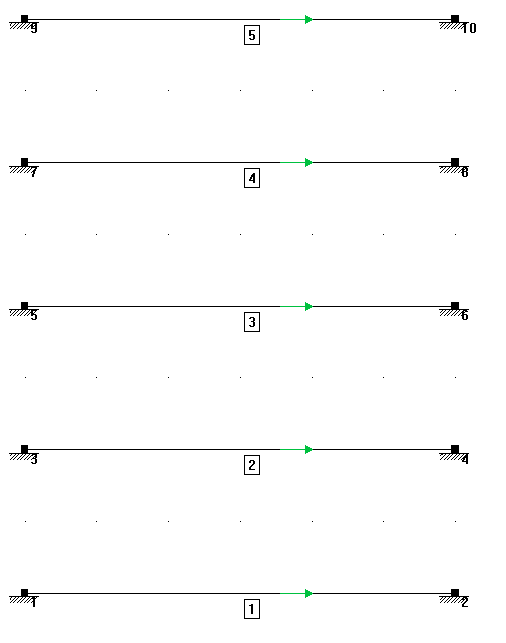With the following results:But what does the elementary case say?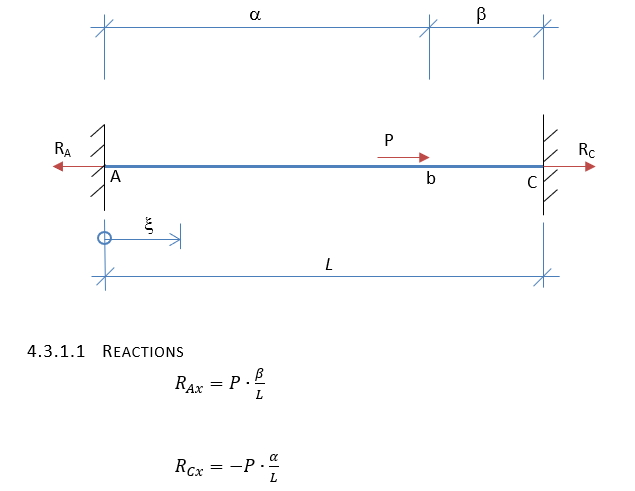So this would calculate to RAx=P*2/6=3.33kN and RCx=-P*4/6=6.67kN.

Does this mean that FEM-Design is wrong?

Well, no...

The items we havn't been looking at are the supports. In FEM-Design they are set with a spring stiffness: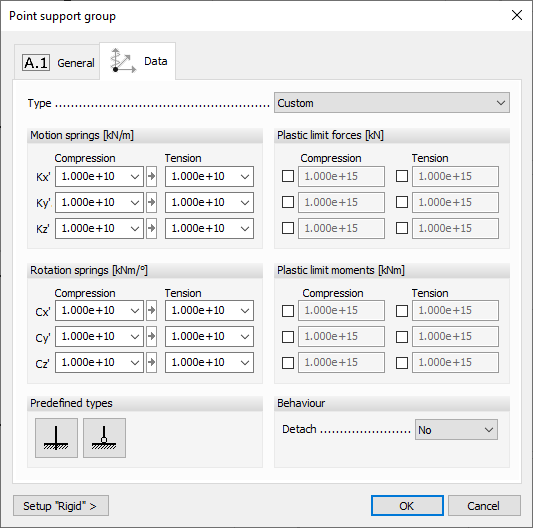In Frame Analysis they are just set as "supports" without any elastic spring-properties: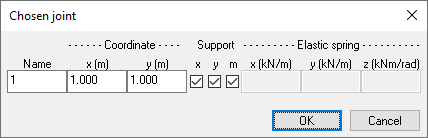The supports in Frame Analysis do not have a stiffness value or spring value but are actually being calculated before the start of the analysis. And the stiffness is set to 100x the stiffness of most stiff object.

So in FEM-Design it is the relative value between the structure (the fictious bar in our case) and the supports (default value: 10^10 kN/m) that cause the differences of the calculations. If we would increase the values of the support stiffnesses higher it would behave similar to a theoretically rigid support, while in Frame Analysis the supports will always be 100 times more stiff, no matter what the stiffness of the elements would be.

It is possible to increase it even higher, up to 10^15 kN/m, but that would be the highest value possible.

The value of the rigidity can be changed here:By increasing the stiffnesses of the supports in FEM-Design (to 1e+15) it is possible to achieve better results, but stiffnesses in these ranges are close to impossible to achieve in real world, only in theory.F
Fredrik is the author of this solution article.

Did you find it helpful? Yes No

Send feedback